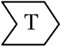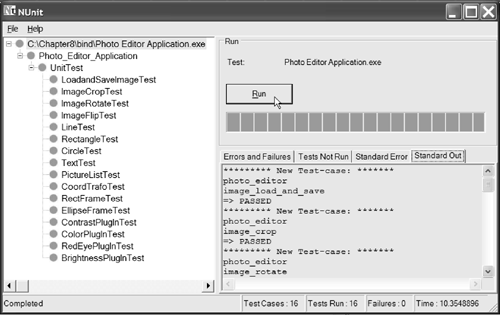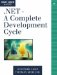# Unit TestsThe unit tests for the functionality implemented in this iteration must prove that the plugins that were developed can be loaded by an application at run time. In addition, the tests should show that the calculation is done correctly.

For image-processing algorithms such as the contrast functionality, it can be very difficult to prove exact correctness. For the unit tests in this chapter, the proof of correctness is to calculate some pixel values and then check them against the expected output. Ideally, the proof should validate that the calculation of all the pixels in the image is correct. In some cases, you can do this by applying a second algorithm that performs the same output by using a different algorithm.

Sometimes it is not possible to have a second algorithm to check the result against. In that case, it's very common to use a gold standard to verify the result of the images. The gold standard is a result that has been defined as correct by experts, and the actual output of the calculation is compared against it. After the standard is defined, the comparison can be made either automatically or manually. In addition, you can use techniques that rely on statistics, such as mean, standard variation, and average, to identify whether the features of the two images are the same or at least similar.

With this knowledge in mind, we implement the tests of the plugin functionality. The first test case we'll look at is the unit test for the contrast plugin.

8.6.1 The Contrast Plugin Unit Test

The strategy to test the contrast plugin is to create an image whose color values are all zero except for the center pixel. The center pixel will have different red, green, and blue values. This enables a simple calculation of the expected result value at the center position. Another advantage of taking only the center pixel is that it provides the ability to prove whether the calculated value was stored in the correct pixel location.

The implementation of the test is shown in Listing 8.13. First, we create an image with the dimensions 100x75 pixels. Then we create an instance of PlugInInterface. After that, we initialize all the image pixels to 0 as color values, except for the center pixel of the image. We set the center pixel color values to Red = 100, Green = 75, and Blue = 50. Now we call the ApplyImageProcessing method, making sure that before calling the method we set the requested plugin name and parameter values. After the calculation is applied, we check the center pixel and the pixel just left of it for correctness. We dispose of the created image, and the test is concluded.

Listing 8.13 The Contrast Plugin Unit Test Implementation

`/// `

/// Test for F:image_contrast_and_color. /// Checks whether the contrast plugin /// can be loaded at run time and whether the /// calculation is correctly performed on the image. ///

/// F:image_contrast_and_color [Test] public void ContrastPlugInTest() { const int width = 100; const int height = 75; Console.WriteLine("image_contrast"); // Create a new bitmap. Bitmap testImage = new Bitmap(width, height); PlugInInterface loader = new PlugInInterface(); // Set all pixels in the image to black (0), // except for the center pixel, which is set to // Red = 100, Green = 75, Blue = 50 for(int i = 0; i < height; ++i) { for(int j = 0; j < width; ++j) { if( i == ((height/2)) && (j == (width/2))) { testImage.SetPixel(j, i, Color.FromArgb(100, 75, 50)); } else { testImage.SetPixel(j, i, Color.FromArgb(0, 0, 0)); } } } loader.TmpBitmap = testImage; loader.SliderName = "Contrast"; loader.Param1 = 1; loader.Param2 = loader.Param3 = 0; // Invoke the ProcessImage method from the // contrast plugin. loader.ApplyImageProcessing(); // Test the center pixel color value for correctness Color pixColor = testImage.GetPixel(width/2, height/2); Assertion.AssertEquals("Center Pixel Red Value", pixColor.R, 255); Assertion.AssertEquals("Center Pixel Green Value", pixColor.G, 243); Assertion.AssertEquals("Center Pixel Blue Value", pixColor.B, 162); // Check whether position on pixel before the centerPixel is 0 // value color. pixColor = testImage.GetPixel(width/2 -1, height/2); Assertion.AssertEquals("Center Pixel Red Value", pixColor.R, 0); Assertion.AssertEquals("Center Pixel Green Value", pixColor.G, 0); Assertion.AssertEquals("Center Pixel Blue Value", pixColor.B, 0); testImage.Dispose(); amIPassed = true; }

## Do It Yourself

Implement other tests to prove the correctness of the calculation performed by the plugin.

Hint: Check an image with uniform pixel values and one with equally distributed color values. Also use various image sizes.

8.6.2 The Color Plugin Unit Test

Testing the color plugin is a straightforward task that includes creating an image with known color values, applying an offset on the color values, and then verifying that the result is correct.

The implementation is shown in Listing 8.14. Start by creating a new PlugInInterface object, and then create a new Bitmap image with the given width and height. Then set each pixel in the image to the values red = 110, green = 99, and blue = 76. Invoke the color plugin, and perform the calculation with the provided color correction values (red = 55, green = 8, blue = 100). After the calculation is finished, check each pixel to determine whether the color values correspond to the sum of the original plus the offset value. If everything is correct, then the test is passed.

Listing 8.14 The Color Plugin Unit Test Implementation

`/// `

/// Test for F:image_contrast_and_color. /// Checks whether the color plugin /// can be loaded at run time and whether the /// calculation is correctly performed on the image. ///

/// F:image_contrast_and_color [Test] public void ColorPlugInTest() { const int width = 125; const int height = 90; Color pixColor; PlugInInterface loader = new PlugInInterface(); Console.WriteLine("image_contrast_and_color"); // Create a new bitmap. Bitmap testImage = new Bitmap(width, height); // Set all pixels in the image to // Red = 110, Green = 99, Blue = 76) for(int i = 0; i < height; ++i) { for(int j = 0; j < width; ++j) { testImage.SetPixel(j, i, Color.FromArgb(110, 99, 76)); } } // Invoke the ProcessImage method from the // color plugin. loader.TmpBitmap = testImage; loader.SliderName = "Color"; loader.Param1 = 55; loader.Param2 = 8; loader.Param3 = 100; loader.ApplyImageProcessing(); // Walk through the entire image for(int i = 0; i < height; ++i) { for(int j = 0; j < width; ++j) { // Check each pixel and color component // for correctness. pixColor = testImage.GetPixel(j, i); Assertion.AssertEquals("Pixel Red Value", pixColor.R, 165); Assertion.AssertEquals("Pixel Green Value", pixColor.G, 107); Assertion.AssertEquals("Pixel Blue Value", pixColor.B, 176); } } testImage.Dispose(); amIPassed = true; }

## Do It Yourself

Implement other tests to prove the correctness of the calculation performed by the plugin.

Hint: Check an image with uniform pixel values and one with equally distributed color values. Also use various image sizes.

8.6.3 The Red Eye Plugin Unit Test

The strategy for testing the red eye plugin is similar to the previously shown test implementations. First, an image is created with known color values. Then the red eye plugin is loaded with the required parameters. Then the result is checked against the expected color values. Listing 8.15 shows the implementation of the unit test.

The implementation of this test is very similar to that of the previous ones. The main difference lies in the calculation of the expected output values. For the red eye tool, only a rectangular part of the image is taken into account in applying the red eye reduction. In the example shown, the area of interest for the red eye tool is between the coordinates x = 50, y = 45 and x = 59, y = 54. Within that area, the tool reduces the red values of the image to 80 percent of their original values.

Listing 8.15 The Red Eye Plugin Unit Test Implementation

`/// `

/// Test for F:image_special_effects. /// Checks whether the red eye plugin /// can be loaded at run time and whether the /// calculation is correctly performed on the image. ///

/// F:image_special_effects [Test] public void RedEyePlugInTest() { const int width = 75; const int height = 90; Color pixColor; PlugInInterface loader = new PlugInInterface(); Console.WriteLine("image_contrast_and_color"); // Create a new bitmap. Bitmap testImage = new Bitmap(width, height); // Set all pixels in the image to // Red = 100, Green = 198, Blue = 55 for(int i = 0; i < height; ++i) { for(int j = 0; j < width; ++j) { testImage.SetPixel(j, i, Color.FromArgb(100, 198, 55)); } } // Invoke the ProcessImage method from the // red eye plugin. loader.TmpBitmap = testImage; loader.SliderName = "RedEye"; loader.Param1 = 50; loader.Param2 = 45; loader.Param3 = 10; loader.ApplyImageProcessing(); // Walk through the entire image for(int i = 0; i < height; ++i) { for(int j = 0; j < width; ++j) { // Check each pixel and color component // for correctness. pixColor = testImage.GetPixel(j, i); if(((j >= 50) && (j < 60)) && ((i >= 45) && (i < 55))) { Assertion.AssertEquals("Pixel Red Value", pixColor.R, 80); } else { Assertion.AssertEquals("Pixel Red Value", pixColor.R, 100); } Assertion.AssertEquals("Pixel Green Value", pixColor.G, 198); Assertion.AssertEquals("Pixel Blue Value", pixColor.B, 55); } } testImage.Dispose(); amIPassed = true; }

Figure 8.10 shows the output of a test run using the NUnit GUI.

Figure 8.10. NUnit Output of Dynamically Loadable Component Test## Do It YourselfImplement other tests to prove the correctness of the calculation performed by the plugin.

In addition, implement some test cases for the brightness plugin. The strategy for testing the brightness functionality is very similar to that of the tests shown for the other plugins. Some tests for the brightness plugin are provided with the distributed source code on the book's CD.

Hint: Check an image with uniform pixel values and one with equally distributed color values. Also use various image sizes..NET-A Complete Development Cycle
ISBN: 0321168828
EAN: 2147483647
Year: 2005
Pages: 123